## Introduction

Choruses of male frogs can be detected in various places, e.g., the streams in rain forests and the edges of ponds1,2,3. The spatial distribution of calling frogs is significantly diverse depending on species and their habitats. Japanese tree frogs (Hyla japonica) are one of the commonest frog species in Japan4. Choruses of male Japanese tree frogs are audible at night mainly along edges of paddy fields, which contain rich water suitable for cultivation of rice, between early spring and late summer. Laboratory experiments have revealed various types of their synchronized calling behavior, e.g., antisynchronization of two individual frogs5 and 1:2 antisynchronization and tri-phase synchronization of three individual frogs6. In choruses at a paddy field, male Japanese tree frogs dynamically change their calling times and positions based on acoustic interactions with other individuals. The spatio-temporal dynamics in such interactive choruses can be mathematically understood as a system of coupled oscillators5,6,7,8. Our motivation in this study is to investigate the spatio-temporal dynamics of their choruses in natural habitats.

Spatio-temporal dynamics in coupled-oscillator systems, collective or not, has been studied both experimentally and theoretically. Experimental studies revealed various types of the spatio-temporal structures in the real world. For instance, the Belousov-Zhabotinsky reaction generated rich examples of pattern formation such as target patterns and spiral waves8,9; experiments on colliding microtubules yielded self-organized structures of large-scale vortex lattices10. Furthermore, theoretical studies elucidated plausible nonlinear mechanisms generating such spatio-temporal dynamics. For example, a phase oscillator model provided a valuable tool describing synchronization phenomena in coupled-oscillator systems8; the phase oscillator model was then extended to include a system consisting of mobile oscillators11,12,13. We previously demonstrated that the synchronized behavior of male Japanese tree frogs observed in laboratory experiments could be qualitatively explained as a system of coupled but not mobile oscillators5,6,7.

On the other hand, the collective behavior of animals, e.g., flashing of fireflies9 and chirping of crickets1, has been attracting a great deal of attention in the light of biology as well as many other disciplines. These animals dynamically change their positions and also interact with each other by using various signals such as bioluminescence and chirping. Consequently, we can expect to observe various spatio-temporal structures in their positions and signals. However, field research of such structures in animal behavior has been restricted because of the difficulties faced in carrying out such research, e.g., in finding where many individuals exist and how they are spatially distributed. Studying the collective behavior of Japanese tree frogs via field research and mathematical modeling could contribute to further understanding of collective animal behavior and could also aid in extending the framework of the phase oscillator model.

## Results

### Numerical simulation of spatio-temporal dynamics in collective frog choruses at a circular field

We model the spatio-temporal dynamics inherent in the calling times and positions of male Japanese tree frogs by using equations (5)(9) that are mentioned in Methods. Based on this model, we theoretically examine organized structures in their choruses at a paddy field. Note that the geometric shape of a paddy field is first assumed to be a circle in this model, for simplicity (Fig. 1). The parameter values of the model are then fixed on the basis of laboratory experiments and field observations. Laboratory experiments have revealed that an isolated male Japanese tree frog calls about 4 times per second5, so that the intrinsic angular velocity ωn in equation (5) is fixed as ωn = 8π rad/s for all the individual frogs. Furthermore, in our field observations, the perimeter of all the edges of a paddy field was typically more than 100 m and more than about 20 individual frogs simultaneously called in one paddy field. Therefore, the radius of the paddy field L and the total number of the male frogs N are fixed as L = 20 m and N = 20, for simplicity. However, since the parameter Knm in equations (7) and (8) is difficult to be estimated from laboratory experiments or field observations, Knm is fixed to be the unit value as Knm = 1, for simplicity.

Figures 2 and 3 show the results of numerical simulation, which are obtained by assuming different initial conditions but the same parameter values ωn = 8π rad/s, L = 20 m, N = 20 and Knm = 1. Frogs are indexed from 1 to N along the edge of the circular field in the counterclockwise direction (Fig. 2A). Our simulation demonstrates that two kinds of spatio-temporal dynamics are bistable depending on the initial conditions (Figs. 2 and 3). The first dynamical structure is shown in Figure 2A and B; whereas the male frogs are positioned along the edge of the field at the same interval (Fig. 2A), each neighboring pair of male frogs synchronize in anti-phase, forming two clusters (Fig. 2B). The second structure is shown in Figure 3A and B; whereas the male frogs are positioned along the edge of the field as well (Fig. 3A), each neighboring pair of male frogs synchronize in almost anti-phase with a spatial phase shift (Fig. 3B); consequently, a wavy state is realized in each cluster, which can be described as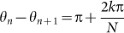by using a nonzero integer k describing the wave number of this state. We name the spatio-temporal dynamics in Figure 2 as two-cluster antisynchronization and that in Figure 3 as wavy antisynchronization.

To detect occurrences of two-cluster and wavy antisynchronization, we introduce the following order parameters Rcluster and Rwavy as the extensions of the order parameter for in-phase synchronization8, i.e.,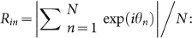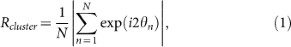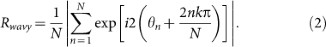Figures 2C and 3C show the time series data of Rcluster, Rwavy and Rin. When two-cluster antisynchronization is realized as shown in Figure 2B, only Rcluster takes a high value around 1 (Fig. 2C). In contrast, when wavy antisynchronization is realized as shown in Figure 3B, only Rwavy for k = 1 reaches a high value around 1 (Fig. 3C). Thus, the order parameters Rcluster and Rwavy can be used to discriminate between two-cluster antisynchronization and wavy antisynchronization. In addition, we perform linear stability analysis by assuming circular distribution of the calling frogs and show that both two-cluster antisynchronization and wavy antisynchronization are asymptotically stable (see Supplementary information). Note that the same parameter values are assumed for the linear stability analysis, i.e., ωn = 8π rad/s, L = 20 m, N = 20 and Knm = 1.

### Numerical simulation of the stability of two-cluster antisynchronization and wavy antisynchronization at a rectangular field

The usual geometric shape of a paddy field is not a circle but a rectangle in Japan. In this section, the stability of two-cluster antisynchronization and wavy antisynchronization is analyzed by replicating the shape of an actual paddy field in our model.

We describe the shape of a rectangular paddy field by using two parameters Lx and Ly, which represent the length and width of the field, respectively (Fig. 4A). In addition, the summation of Lx and Ly is constrained as Lx + Ly = 60 m, for consistency with the perimeter of the circular field shown in Figures 2A and 3A. Then, Gn(rn) in equation (6) is defined as follows: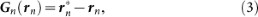where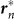represents the vector from the origin 0 to the point on the edges that is nearest to the position of the nth frog rn, as shown in Figure 4A. We use the term Gn(rn) in equation (3), which changes its sign across the boundary condition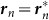, to explain the attraction of the male frogs towards the edges of the field.

Figure 4B shows the results of numerical simulation based on the assumption of ωn = 8π rad/s, N = 20, Knm = 1 and Lx + Ly = 60 m in the present mathematical model of equations (3) and (5)(8). In this simulation, the parameters Lx and Ly are varied with an interval of 2 m in the ranges 30 ≤ Lx ≤ 60 and 0 ≤ Ly ≤ 30 under the constraint Lx + Ly = 60. For each parameter set of Lx and Ly, occurrences of two-cluster antisynchronization and wavy antisynchronization are calculated for 500 runs of the simulation with different initial conditions: namely, if only Rcluster is more than 0.9 at t = 30000, the dynamics is considered as two-cluster antisynchronization; if only Rwavy is more than 0.9 for one of k = −4, −3, −2, −1, 1, 2, 3 and 4 at t = 30000, the dynamics is considered as wavy antisynchronization. As shown in Figure 4B, two-cluster antisynchronization is more frequently observed than wavy antisynchronization, except for the cases of (Lx, Ly) = (52, 8), (54, 6) and (56, 4).

When (Lx, Ly) = (52, 8), (54, 6) and (56, 4), both two-cluster antisynchronization and wavy antisynchronization are not or very rarely detected (Fig. 4B). Why does such instability occur at these parameter values? Figure 5A and B represents an example of the spatio-temporal dynamics at (Lx, Ly) = (56, 4). As shown in Figure 5A, almost equilaterally triangular patterns are realized in many frog trios because of the narrow and long geometric shape of the rectangular field characterized by (Lx, Ly) = (56, 4). Since the coupling strength of Γnmm − θn, rmrn) in equation (7) depends on the distance between frogs, such equilateral-triangle structures cause the frog trios to interact with almost the same strength. Moreover, Γnmm − θn, rmrn) in equation (7) is assumed to be a sinusoidal function. It has been theoretically shown that almost the same coupling strength with the sinusoidal function, e.g.,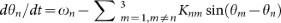with n = 1, 2, 3, ωn = ω and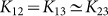, can strongly frustrate the calling behavior of three frogs7. We speculate that such frustration is the source of the instability of two-cluster and wavy antisynchronization at (Lx, Ly) = (52, 8), (54, 6) and (56, 4). In fact, a snapshot of the phases at t = 20000 does not show any organized structure such as the two-cluster and wavy antisynchronization (Fig. 5B) and all the order parameters Rcluster, Rwavy and Rin take considerably less values than 1 (Fig. 5C).

Thus, our numerical simulation of the present mathematical model suggests that the two-cluster antisynchronization of the male frogs can be more frequently observed than wavy antisynchronization at a rectangular paddy field.

### Field research on collective choruses of male Japanese tree frogs

Figure 7A shows the time series data of the light pattern of sound-imaging devices14 deployed at an actual paddy field (see Methods and Fig. 6), capturing the chorus structures of male Japanese tree frogs. The colored plots represent the light intensity of each device, which has been calculated by subtracting the average light intensity of each device that can slightly vary depending on its tuning14; an index is attached to each device from one end of the edge, which is closer to the camera, to the other end (Fig. 6C). The device nearest to each calling frog was estimated every 15 sec, by analyzing the summation of the light intensity of the deployed devices: namely, if the summation at one device exhibited a local peak and exceeded a threshold, the device was determined to be nearest to one calling frog. Through this analysis, the threshold was set as 3 × 29.97 fps (frames per second) × 15 sec. To estimate the calling times of each frog, the light pattern of the device nearest to each calling frog was then analyzed: namely, when the light pattern of the device exceeded a threshold, the corresponding times were detected as the calling times of the frog (see supplemental materials of the reference 6). In this analysis, 50% of the maximum light intensity of respective devices was used as a threshold value.

Figure 7B shows the calling times of 6 frogs obtained from the light pattern shown in Figure 7A. We observed that some pairs called synchronously. For instance, the 2nd and 3rd frogs stably synchronized in almost anti-phase. The calling times of the nth frog were then described by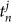, where j represented the jth calling and the phase of the nth frog at time t was evaluated as follows6,9: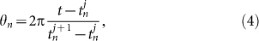where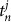and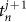described the times of the two calls covering time t. We did not calculate the phase in the case of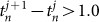or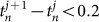, because the inter-call intervals of a single Japanese tree frog were around 0.25 sec5. Subsequently, the order parameters Rcluster, Rwavy and Rin were estimated during the collective choruses of more than 3 frogs, by substituting the time series data of θn to equations (1) and (2) and to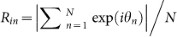8. Figure 7C represents the time series data of Rcluster, Rwavy and Rin. It was shown that Rcluster stably took considerably larger values than Rwavy and Rin, which meant that two-cluster antisynchronization was realized in this chorus of the 6 male frogs. In addition to the data shown in Figure 7 that were recorded on 15th June in 2011, we analyzed the data recorded on 12th, 14th, 16th and 17th June in 2011 and calculated the order parameters of Rcluster, Rwavy and Rin. It should be noted that we did not analyze the data recorded on 11th June in 2011 (see Methods), since the data were too complicated to precisely estimate the positions of calling frogs because of the presence of a very large number of calling frogs (Fig.S7 in Supplementary information).

To examine the reproducibility of two-cluster antisynchronization, we performed two kinds of analyses. First, the mean values of the order parameters were calculated as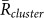,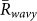for k = 1 and −1 and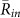, from the 15 min video data of the 5 field observations (Table 1A). It was shown that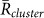was larger thanfor k = 1 and −1 andin all the data. Second, the ratio of each state was calculated from the same data (Table 1B), by estimating the total duration when the order parameters exceeded a threshold value of 0.8. This analysis demonstrated that the ratio of two-cluster antisynchronization was considerably larger than the ratios of the other states. Thus, two-cluster antisynchronization of male Japanese tree frogs was more frequently observed at an actual paddy field.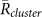Table 1 Stability analyses of synchronization states on the basis of the 5 field observations. In this table, Data (1)–(5) correspond to the 15 min video data recorded on 12th, 14th, 15th, 16th and 17th June in 2011, respectively. Note that we did not use the data recorded on 11th June in 2011, since the data were too complicated to precisely estimate the positions of calling frogs because of the presence of a very large number of calling frogs at the paddy field (Fig.S7 in Supplementary information). (A) The averaged order parameters of two-cluster antisynchronization, in-phase synchronization and wavy antisynchronization. The total duration of choruses including more than 3 frogs were 11.5 min, 1.7 min, 5.2 min, 0.9 min and 12.34 min, respectively. By using these data sets of the choruses, we estimated the mean values of the order parameters as , and . It was shown that was larger than for k = 1 and −1 and in all the field observations. (B) Ratios of two-cluster antisynchronization, in-phase synchronization and wavy antisynchronization. In this analysis, we summed up the times when each order parameter exceeded a threshold value of 0.8 and then divided the duration of each state by the total chorus duration of more than 3 frogs. The ratio of two-cluster antisynchronization was considerably larger than those of in-phase synchronization and wavy antisynchronization

## Discussion

This paper presents theoretical and experimental studies on spatio-temporal dynamics in collective frog choruses. First, a mathematical model is proposed to describe the nonlinear dynamics inherent in the calling times and positions of male Japanese tree frogs. The numerical simulation shows that organized spatio-temporal dynamics, i.e., two-cluster antisynchronization and wavy antisynchronization, are realized in the mathematical model (Figs. 2 and 3). Second, the stability of two-cluster and wavy antisynchronization is analyzed by numerically varying the length parameters of a rectangular paddy field. It is demonstrated that two-cluster antisynchronization is more frequently observed than wavy antisynchronization (Fig. 4B). Finally, field research was performed on actual choruses of male Japanese tree frogs at a paddy field by using our sound-imaging method14. Analysis of the recorded video showed that two-cluster antisynchronization was more frequently detected in choruses of male Japanese tree frogs (Fig. 7 and Table 1). Thus, the present mathematical model qualitatively explains the result of the field research, i.e., the existence of two-cluster antisynchronization in the choruses of male Japanese tree frogs.

As for the spatial structures in frog choruses, our field research revealed that calling frogs were sparsely positioned along an edge of a paddy field at an interval ranging from 0.8 to 3.2 m (Fig. 7B), using the spatial resolution by the devices deployed at intervals of 40 cm. In general, most frog species can be classified as either explosive or prolonged breeders2,15. Experimental studies have shown that, whereas explosive breeders exhibit considerably denser distribution in their breeding site, prolonged breeders exhibit sparser distribution2,15. Since Japanese tree frogs are classified as prolonged breeders4,15, their sparse spatial distribution revealed by our field research can be well understood on the basis of their breeding type. Moreover, as shown in Figures 2A, 3A and 5A, our mathematical model qualitatively explains the sparse distribution of male Japanese tree frogs according to the competitive interaction terms described by equations (7) and (8).

As for the spatio-temporal dynamics in frog choruses, wavy antisynchronization was rarely detected in the field research, although it appeared as a stable solution in the numerical simulation (see Figs. 3 and 4B). This inconsistency may be explained by a specific field condition observed during our recordings. At the paddy field shown in Figure 6C, the sound-imaging devices were deployed along one edge of the field where a considerably larger number of male frogs aggregated than along the other 3 edges of the field (see Methods). Therefore, the calling frogs mainly aligned along the one edge covered by our recording system and then straight-line distribution of the male frogs was approximately realized at the paddy field. Our numerical simulation suggests that, in such straight-line distribution of the male frogs, wavy antisynchronization is not observed and only two-cluster antisynchronization is observed (see the case of (Lx, Ly) = (60, 0) in Fig. 4B). Furthermore, linear stability analysis has shown that two-cluster antisynchronization is asymptotically stable for the straight-line distribution (Fig.S2C in Supplementary information).

From the mathematical point of view, it is an important future problem to examine the stability of two-cluster and wavy antisynchronization by varying the parameter values in the present mathematical model. In particular, we expect that the stability can be affected by the number of the male frogs, N, which is fixed as N = 20 in this study. For example, exact two-cluster antisynchronization cannot exist for circular distribution with odd values of N, because of frustration that all the neighboring pairs cannot synchronize in anti-phase7. In contrast, two-cluster antisynchronization for the straight-line distribution can remain as a stable equilibrium state even for odd values of N, because all the neighboring pairs along the straight line can synchronize in anti-phase as θn − θn+1 = π (mod 2π), for n = 1, 2, …, N − 1.

As shown in Figure 7C, the order parameters Rcluster, Rwavy and Rin showed complex dynamics during the recording at the paddy field. This result suggests that male Japanese tree frogs dynamically change their calling times even in the same chorus beyond the expectation of the present mathematical model. Further studies are required to derive a mathematical model explaining such complex dynamics observed in an actual field. For the modification of the present mathematical model, it is useful to estimate three functions, Γnmm − θn, rmrn), Fnmm − θn, rmrn) and Gn(rn), on the basis of laboratory experiments and field research. The functions Γnmm − θn, rmrn) and Fnmm − θn, rmrn) may be estimated by laboratory experiments. For instance, recording of two calling frogs that hop around a room can be used to represent fundamental data to study the interactions between two individuals. Subsequently, Γnmm − θn, rmrn) may be estimated according to the method provided in the reference 16 that makes it possible to calculate the interaction terms in the phase oscillator model from the desynchronized time series data of two interacting elements. However, it seems to be difficult to experimentally determine Gn(rn), because male Japanese tree frogs move to a paddy field without emitting calls according to our field observations. A sound-imaging method cannot be applied to such silent frogs and other tracking methods need to be developed.

Advertisement calls of male frogs have two roles, i.e., attracting conspecific females and defending their own territories2. In this section, the behavioral meanings of two-cluster antisynchronization are discussed based on these two roles of advertisement calls. As regards the first role of attracting conspecific females, two-cluster antisynchronization of male frogs can strengthen the sound-pressure level of their chorus, because male frogs involved in the same cluster call synchronously almost in-phase. Such a collective chorus with a high sound-pressure level may attract more females far from the chorus site. After the females arrive at the breeding site, the males must compete against each other to mate with one of the females. In this final step, the calling properties of individual male frogs, e.g., sound-pressure level and frequency, can be also important for attracting females2, because such calling properties can include information about body sizes and physical conditions of the male frogs. As regards the second role of defending their territories, Figure 7B has demonstrated that neighboring pairs of male frogs, e.g., the pair of the 2nd and 3rd frogs and the pair of the 5th and 6th frogs, tend to call alternately in anti-phase. Such antisynchronization between neighboring males can help them to claim their own territories each other because of a small amount of call overlap5,6,17. If neighboring pairs of male frogs synchronize in anti-phase respectively, two-cluster antisynchronization is automatically realized. Therefore, antisynchronization of neighboring pairs can be the origin of global two-cluster antisynchronization. However, in-phase synchronization between a neighboring pair was also detected. For example, the pair of the 1st and 2nd frogs synchronized almost in-phase (Fig. 7B). The role of such cooperative behavior is still unknown and needs to be further examined.

The present mathematical model can be applied to theoretical studies on the collective behavior of other species of animals. For instance, several species of insects, such as fireflies, crickets and cicadas, interact with each other by periodically emitting signals involving lights and sounds9. The nonlinear dynamics in the collective behavior of such animals can be examined on the basis of our mathematical model, by varying the interaction terms Γnmm − θn, rmrn) and Fnmm − θn, rmrn) and the geometric shape of their habitats. In addition, when the target animals are nocturnal and interact through sounds, our sound-imaging method can be similarly applied to detect their calling times and positions14. However, it should be noted that the present method has several limitations. One of the limitations is that we had to manually tune the gain of each Firefly14 and then the response of each Firefly to the same sound could have been slightly different. Such a difference in the tuning can cause the problem that the position of a single frog is detected twice. For example, in the data of Figure S4 shown in Supplementary information, two close peaks at the 31st and 33rd devices were detected as the positions of calling frogs, respectively. In addition, the calling times estimated from the light patterns of the two devices were almost the same. We speculate that these signals of the 31st and 33rd devices originated from the calls of a single frog, since the 32nd device weakly responded to the calls because of bad device tuning. In this study, we carefully chose suitable threshold values in the data analysis to avoid this problem. However, further studies to improve the device performance and data analysis method are required.

## Methods

### Mathematical modeling of collective frog choruses

To theoretically examine the spatio-temporal structures realized in the choruses of male Japanese tree frogs, we propose a mathematical model describing their behavior in an actual field. Whereas a single male Japanese tree frog calls periodically, a pair of the male frogs interact through sounds5,6. In addition, the male frogs hop around fields in natural habitats. We model such dynamics in calling times and positions of the male frogs originating from their acoustic interactions, as follows (Fig. 1):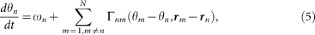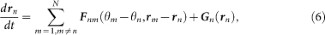where θn (n = 1, 2, …, N) represents the phase of the calling of the nth frog5,6,7 and the vector rn represents the position of the nth frog in a two-dimensional space. The parameter ωn represents the angular velocity of the calling of the nth frog. It is then assumed that θn = 0 (mod 2π) corresponds to the timing of the calls emitted by the nth frog, so as to explain the periodic calling behavior of the isolated male frogs5,6. The functions Γnmm − θn, rmrn) and Fnmm − θn, rmrn) (n, m = 1, 2, …, N and nm) represent the effects from the mth frog to the nth frog. By simply extending the phase oscillator model described by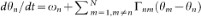8, Γnmm − θn, rmrn) and Fnmm − θn, rmrn) are defined as 2π-periodic functions of the phase difference, θm − θn and also functions of the relative position between the nth and mth frogs, rmrn. The function Gn(rn) is used to explain our field observations that male Japanese tree frogs aggregate along the edges of paddy fields in natural habitats14. Moreover, the geometric shape of the field is first assumed to be a circle, for simplicity; the radius of the field is described by the positive constant L and the center of the field is set at the origin 0 in the two-dimensional space (Fig. 1).

Then, we determine the three functions Γnmm − θn, rmrn), Fnmm − θn, rmrn) and Gn(rn) in equations (5) and (6), based on experimental results and field observations of the behavior of male Japanese tree frogs.

Laboratory experiments revealed that two individuals of male Japanese tree frogs, which were placed in small cages respectively, called alternately almost in anti-phase π5,6. To qualitatively explain this antisynchronization (or call alternation) unique to male Japanese tree frogs, we used the phase oscillator model with a simple sinusoidal function7, i.e.,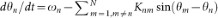. By extending the model, we define Γnmm − θn, rmrn) in equation (5) as follows: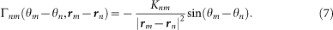Because the male frogs interact through sounds that decay proportional to the inverse of the square of their distance, Γnmm − θn, rmrn) is assumed to be a function of 1/|rmrn|2. The parameter Knm represents a positive symmetrical coupling coefficient between the nth and mth frogs, i.e., Knm = Kmn.

The calling behavior of male Japanese tree frogs studied here is classified as advertisement calls. In general, advertisement calls of male frogs have two roles of attracting conspecific females and also claiming their own territories to other conspecific males2. The important point is that, during antisynchronization of two individuals, information included in calls of one individual is not masked by calls of the other individual because of a small amount of call overlap17. These two properties of advertisement calls and call overlap suggest that antisynchronization is capable of helping each pair of male frogs to mutually interact and then robustly defend their own territories5,6. To model this conjecture about antisynchronization, Fnmm − θn, rmrn) in equation (6) is defined as follows: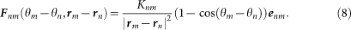Here, Fnmm − θn, rmrn) is assumed to include the factor Knm/|rmrn|2 as in the case of Γnmm − θn, rmrn), because Fnmm − θn, rmrn) also models the interaction of the male frogs via calling sounds. Moreover, Fnmm − θn, rmrn) is assumed to include the factor (1 − cos(θm − θn))enm, where enm is a unit vector between rn and rm, i.e.,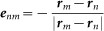. In this term, 1 − cos(θm − θn) takes the maximum positive value at θm − θn = π, which means that the nth and mth frogs attempt to move towards the opposite directions during antisynchronization; 1 − cos(θm − θn) takes the minimum value of 0 at θm − θn = 0, which means that the nth and mth frogs do not affect their positions each other during in-phase synchronization. It should be noted that male frogs face difficulties in their acoustic interaction during in-phase synchronization because of a large amount of call overlap.

We previously observed that male Japanese tree frogs aggregated along the edges of paddy fields14. To explain such localized spatial distribution of male Japanese tree frogs, Gn(rn) is defined as follows: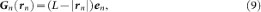where en represents a unit vector between the position of the nth frog rn and the center of the paddy field 0, i.e.,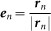. Furthermore, Gn(rn) is assumed to include the factor L − |rn|. Here, L − |rn| is negative when the nth frog is positioned outside the circular paddy field and then the frog is attracted to the edge of the field; on the other hand, L − |rn| is positive when the nth frog is positioned inside the circular paddy field and the frog is attracted to the edge of the field as well. Equation (3) of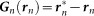is used instead of equation (9) for a rectangular paddy field.

### Recording of frog choruses at an actual paddy field

To test the hypothesis of our numerical simulation suggesting that two-cluster antisynchronization can be frequently observed in the choruses of male Japanese tree frogs (Fig. 4B), the positions and calling times of individual frogs must be obtained. For this purpose, we used a sound-imaging method14. The imaging method is based on the device named Firefly consisting of a microphone and a light emitting diode (LED)14 (Fig. 6B); the LED of the Firefly unit is illuminated, when capturing nearby sounds. Along one edge of a paddy field where a considerably larger number of male Japanese tree frogs were calling than along the other three edges, 85 or 86 devices were deployed at intervals of 40 cm (Fig. 6C); the illumination pattern of the devices was recorded by a Sony video camera (HDR- XR550V, 29.97 fps). Note that the lights of some devices were not detected, when those devices were deployed far from the camera and were not illuminated by frog calls. We carefully checked all the data and confirmed that the lights of at least 40 devices close to the camera were stably captured even when those devices were not strongly illuminated by frog calls. Hence, we used the light patterns of 40 devices close to the camera for data analysis. An index was attached to each device from one end of the edge, which was closer to the camera, to the other end.

Recordings were carried out between 20:00 h and 24:00 h on 11th, 12th, 14th, 15th, 16th and 17th June, 2011, in Oki island, Shimane, Japan. The ambient temperature ranged between 15°C and 21.5°C and the humidity ranged between 49% and 93%. The data corresponding to the first 15 min of all the recorded video were then divided into dozens of pictures. All the field observations in this study were performed in accordance with the guidelines approved by the Wako Animal Experiments Committee of RIKEN and the Animal Experimental Committee of Kyoto University.